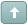Error

 Previous Topic Next Topic
 Patrick #1 Posted : Tuesday, November 2, 2004 5:21:23 PM(UTC)Rank: Advanced MemberGroups: Registered, Registered Users, SubscribersJoined: 9/8/2004(UTC)Posts: 2,266Was thanked: 1 time(s) in 1 post(s) The MetaStock Formula for MACD is:Mov(C,12,E)-Mov(C,26,E)Note that here we use periods 12 and 26 and not a percentage value.The formula to calculate the number of periods for an Exponential Moving Average from an exponential average percentage value is as follows:% = 2 / (t+1)t = the periods of the EMAThe following is an example of how to solve for “t”, using a value of 10% or 0.1:0.1 = 2 / (t+1) 0.1 (t+1) = 2 (t+1) = 2 / 0.1 or 20 t = 19So for 12 we are using : Percentage=2/(12+1)Percentage=2/13Percentage=0.1538461We round this number to say 15% or 0.15For 26 we have 0.075Now let's assume we decompose the MACD formula using these values.Here is what it would look like:Our first average is : Mov(C,12,E)Which is : (C*0.15)+(Prev*(1-0.15))(C*.15)+(prev*.85)Our second average is : Mov(C,26,E)Which is : (C*0.075)+(prev*(1-0.075))(C*.075)+(prev*.925)MA1:=(C*.15)+(prev*.85);MA2:=(C*.075)+(prev*.925);Ma1-MA2Wanna join the discussion?! Login to your Discussions forum accountor Register a new forum account.Users browsing this topic
Forum Jump
You cannot post new topics in this forum.
You cannot reply to topics in this forum.
You cannot delete your posts in this forum.
You cannot edit your posts in this forum.
You cannot create polls in this forum.
You cannot vote in polls in this forum.